• 2022-03-15 11:02:06

一、实验要求

在计算机上验证和测试多层神经网络的原理和算法实现，测试多层神经网络的训练效果，同时查阅相关资料。

• 实验目的

1、掌握多层神经网络的基本原理；

2、掌握多层神经网络的算法过程；

3、掌握反向传播的算法过程；

三、实验内容

实验步骤

1. 请阅读和测试多层神经网络类代码，观察多层神经网络训练过程和结果，请对隐藏层Dense类和多层神经网络MLPClassifier类的代码进行注释，然后代码截图。

1. 请对多层神经网络改进，实现对如下数据的分类。

1. 增加隐藏层神经元个数
 神经元个数 层数为2层 2 10 100 1000

1. 增加隐藏层的层数

手写增加函数层数

调用sklearn自带函数

 隐藏层数 神经元个数5 10 100 500

1. 请实现ReLU激活函数类，与Sigmoid激活函数类，针对同样二分类问题训练的区别。

在相同两层5个神经元下对比

 Sigmoid relu 分类效果 训练速度

结论：

Sigmoid 函数执行指数运算，计算机运行得较慢。

Relu下降速度快但是会出现一些神经元消失的情况，因为导数恒为0

四、实验总结

1权值和偏置已经是被训练好了。对于一个新的输入，进行这样的过程，就能够得到一个预测。那么你就能够通过这个预测来解决一些问题了。所以有机器学习思想的话，这些概念是很容易理解的。而我们的任务就是找到权值和偏置这些参数的值，使得输出的东西让我们满意，达到我们的要求。Sigmoid平滑能够使得权值和偏置非常微小的变动能够使得最终的结果也产生非常微小的变动。机器学习 人工智能
更多相关内容
• MNIST手写数字识别问题的多层神经网络模型实践 按课程案例，动手完成编码实践。 自行设计一种神经网络模型，并尝试采用不同超参数，让模型的准确率达到97.5%。 提交要求： 1、你认为最优的一次带运行结果的源代码...
• 采用基于片上网络通信的多核处理器，设计并实现了一种具有可重构性，运行时配置和更新，以及具有学习能力的多层感知器神经网络结构以及对应的编程模型，并在FPGA上进行了验证。实验结果表明所设计的结构满足精度和...
• 实现一个多层的BP神经网络，将处理线性不可分二分类处理问题是在一个正方形内划一个圆分为两部分进行分类 具有保存参数的功能。每层的个数可设定，层数固定。
• 第七章多层神经网络设计北京工业大学 第七章多层神经网络设计 神经网络的性能主要取决于神经元类型神经网络结构及相应的学习算法因此可以说神经网络的设计其实质就是如何选取神经元及其连接形式如何选择学习算法...
• 多层神经网络1. 回顾2. 多层神经网络结构 1. 回顾 在上一讲中，我们提到从上个世纪80年代开始，人工神经网络领域迎来了新的突破，产生了多层神经网络，人们终于可以利用人工神经网络处理非线性问题了。 2. 多层神经...

# 1. 回顾

在上一讲中，我们提到从上个世纪80年代开始，人工神经网络领域迎来了新的突破，产生了多层神经网络，人们终于可以利用人工神经网络处理非线性问题了。

# 2. 多层神经网络结构

## 2.1 两层神经网络例子

这一讲主要介绍多层神经网络的结构。下面这幅图是最简单的多层神经网络，它包含2层，总共由3个神经元相互连接而成。图1 两层神经网络的例子

输入 X X 向量，有两个分量 x 1 x_1 x 2 x_2 ，输出 y y 是一个数值。我们逐层写出输入到输出之间的关系：
a 1 = ω 11 x 1 + ω 12 x 2 + b 1 a_1=ω_{11}x_1+ω_{12}x_2+b_1 （第一个神经元）
a 2 = ω 21 x 1 + ω 22 x 2 + b 2 a_2=ω_{21}x_1+ω_{22}x_2+b_2 （第二个神经元）
z 1 = φ ( a 1 ) z_1=φ(a_1) （非线性函数）
z 2 = φ ( a 2 ) z_2=φ(a_2) （非线性函数）
y = ω 1 z 1 + ω 2 z 2 + b 3 y=ω_1z_1+ω_2z_2+b_3 （第三个神经元）

当然，我们也可以用一个非常复杂的公式描述多层神经网络输入与输出的关系
y = w 1 φ ( ω 11 x 1 + ω 12 x 2 + b 1 ) + w 2 φ ( ω 21 x 1 + ω 22 x 2 + b 2 ) + b 3 (1) y=w_1φ(ω_{11}x_1+ω_{12}x_2+b_1)+w_2φ(ω_{21}x_1+ω_{22}x_2+b_2)+b_3\tag{1}

这个神经网络分成了两层，第一层是前2个神经元，第二层是后一个神经元，两层之间用非线性函数 φ ( ∗ ) φ(*) 连接起来。

这里有个问题请大家思考一下，在这个多层神经网络模型中，待求的参数是哪些呢

显然，待求的参数有9个，分别是：

• 第一层网络中的（ w 11 ， w 12 ， w 21 ， w 22 ， b 1 ， b 2 w_{11}，w_{12}，w_{21}，w_{22}，b_1，b_2
• 第二层网络中的（ w 1 ， w 2 ， b 3 w_1，w_2，b_3

## 2.2 非线性函数的作用

需要强调的是两层之间的非线性函数 φ ( ∗ ) φ(*) 是必须的。我们不妨考虑一下，如果我们不加这个非线性函数 φ ( ∗ ) φ(*) ，而是让第一层的输出直接作用到第二层的输入上会有什么结果呢？

这时输出 y y 将等于如下这个式子
y = w 1 ( ω 11 x 1 + ω 12 x 2 + b 1 ) + w 2 ( ω 21 x 1 + ω 22 x 2 + b 2 ) + b 3 y=w_1(ω_{11}x_1+ω_{12}x_2+b_1)+w_2(ω_{21}x_1+ω_{22}x_2+b_2)+b_3
把它经过一系列的化简后
y = ( w 1 ω 11 + w 2 ω 21 ) x 1 + ( w 1 ω 12 + w 2 ω 22 ) x 2 + ( w 1 b 1 + w 2 b 2 + b 3 ) (2) y=(w_1ω_{11}+w_2ω_{21})x_1+(w_1ω_{12}+w_2ω_{22})x_2+(w_1b_1+w_2b_2+b_3)\tag{2}
可以看到， y y x 1 x_1 x 2 x_2 加权求和再加上偏置的形式，输出仍然是输入的线性加权求和再加偏置的形式。

如果我们现在假设一个神经元的状况图2 单个神经元的例子

那么将有
y = w 1 x 1 + w 2 x 2 + b (3) y=w_1x_1+w_2x_2+b\tag{3}

综合上述复杂的式(2)及简单的式(3)，我们可以看到，如果式(3)的 w 1 = w 1 ω 11 + w 2 ω 21 w_1=w_1ω_{11}+w_2ω_{21} ，同时另外两个相应的式子也相应的相等，那么式(2)与式(3)将会是同一个模型。也就是说，如果层与层之间不加非线性函数，那么多层神经网络将会退化到一个神经元的感知器模型状态。

我们论证了在多层神经网络中必须加入非线性函数，下一个问题是我们要加的非线性函数是什么呢 ？

这里先直接给出答案，非线性函数是阶跃函数。例如
φ ( x ) = { 1 , x > 0 0 , x < 0 φ(x)=\begin{cases} 1, & x>0\\ 0, & x<0 \end{cases}图3 阶跃函数

为什么要用阶跃函数作为多层神经网络的非线性函数呢？

因为有一个定理，如果非线性函数采用阶跃函数，那么三层神经网络可以模拟任意的非线性函数

# 3. 三层神经网络可以模拟任意的非线性函数

在证明这个定理之前，我们来看一下多层神经网络的结构。我们可以任意的增加神经网络的层数，同时对于每一层我们可以任意的设置这一层神经元的个数，运用前面讲述的连接方式，我们将获得更大的神经网络。例如在如下图4所示的神经网络中，总共有3层，第一层有3个神经元，第二层有2个神经元，第三层有2个神经元，层与层之间用非线性函数连接。图4 多层神经网络结构

接下来我们可以给出3层神经网络模拟任意非线性函数的证明了。

在这里我们假设特征空间是二维的，同时我们假设是二分类问题。对于特征空间大于二维，类别数大于两类的情况，证明的思路也是一样的。

## 3.1 构造三角形非线性函数的神经网络结构

首先，我们来看一个简单的非线性函数。在特征空间上，有一个三角形，在三角形内部的区域属于一类，而在三角形外部的区域属于另一类，如下图所示。我们要用多层神经网络来构造一个函数使得：
如果 x x 在三角形内部，则输出 y > 0 y>0 ;
如果 x x 在三角形外部，则输出 y < 0 y<0

怎么做呢？首先我们假定这个三角形三条边的方程分别是
{ w 11 x 1 + w 12 x 2 + b 1 = 0 w 21 x 1 + w 22 x 2 + b 2 = 0 w 31 x 1 + w 32 x 2 + b 3 = 0 \begin{cases} w_{11}x_1+w_{12}x_2+b_1=0\\ w_{21}x_1+w_{22}x_2+b_2=0\\ w_{31}x_1+w_{32}x_2+b_3=0 \end{cases}图5 三角形非线性函数

同时我们假定上述三个方程中，在朝向三角形的一侧，其方程的值大于0；而远离三角形的一侧，其方程的值小于0。这是一定可以做到的，因为如果做不到，只需要将 w w b b 取相反数即可。

那么我们如何构造一个两层的神经网络来实现在三角形内输出大于0而三角形外输出小于0呢？

构造如下：图6 构造三角形非线性函数神经网络模型

第一层有3个神经元，每个神经元的 w w b b 的值分别对应着三角形每一条边的方程，如下图所示。这样如果一个二维向量 X X ，它的两个分量 x 1 x_1 x 2 x_2 在三角形里面，那么三个神经元输出的 a 1 a_1 a 2 a_2 a 3 a_3 将都大于0经过阶跃函数过后，输出 Z 1 ， Z 2 ， Z 3 Z_1，Z_2，Z_3 都将等于1
φ ( x ) = { 1 , x > 0 0 , x < 0 φ(x)=\begin{cases} 1, & x>0\\ 0, & x<0 \end{cases}另一方面，如果 X X 在三角形外，那么 a 1 a_1 a 2 a_2 a 3 a_3 至少有一个小于0。经过阶跃函数过后， Z 1 ， Z 2 ， Z 3 Z_1，Z_2，Z_3 也至少有一个等于0根据上面的分析，我们在第2层，将 Z 1 ， Z 2 ， Z 3 Z_1，Z_2，Z_3 对应的 w w 全设置为1，而将偏置 b b 设置为-2.5，则满足条件。因为当且仅当 X X 在三角形内， Z 1 ， Z 2 ， Z 3 Z_1，Z_2，Z_3 全为1，经过第2层神经元后，输出为y=0.5；而如果 X X 在三角形外， Z 1 ， Z 2 ， Z 3 Z_1，Z_2，Z_3 不能全为1，至少有一个为0，经过第2层后，输出的y将会是一个负数，这样就达到了模拟这个非线性函数的目的。

## 3.2 构造四边形非线性函数的神经网络结构

下面我们假设特征空间有一个四边形，四边形里面属于一类，而四边形外面属于另一类，如下图所示。我们如何做一个两层神经网络来区分这两类呢？有了前面的例子，这个问题很简单，只需要

• 在第一层增加一个神经元
• 第二层所有权重设置为1
• 偏置设置为-3.5

即可满足条件，可自行验证一下。

那么对于任意的多边形都可以采用这样的方法，所以两层神经网络足以模拟这样多边形的非线性函数。

## 3.3 构造不规则封闭曲线非线性函数的神经网络结构

接下来，如果有一个不规则的封闭曲线呢？例如下图所示，在圆圈里面是属于一类，而圆圈外面属于另一类。我们能否用两层神经网络来模拟这样的非线性函数呢？虽然我们不能精确的获得这个非线性函数，但是我们却可以无限地逼近它。因为任何一个不规则的封闭曲线都可以用多边形去近似。例如我们可以用下面的绿色多边形去近似图上的圆圈，当多边形的边数趋近无限时，第二层神经元的个数也会趋于无限，这时我们可以以任意精度去逼近这个非线性函数。## 3.4 构造两个三角形非线性函数的神经结构

最后我们看一下，如果特征空间有2个三角形，如果 X X 处于两个三角形的任何一个当中，我们把它判定为同一类，而两个三角形的外面是另外一类。这时我们能否用多层神经网络来模拟这个非线性函数呢？

答案是也是可以的。不过对于这个例子，我们需要用3层神经网络来模拟，如图所示。这个神经网络前面两层的设置都已经讲过，我们主要看最后一层，经过简单的推理可以得出，如果 X X 在第一个三角形里面，那么 C 1 = 1 ， C 2 = 0 C_1=1，C_2=0 ；如果 X X 在第二个三角形里面，那么 C 1 = 0 ， C 2 = 1 C_1=0，C_2=1 ；如果 X X 同时在两个三角形外面，那么 C 1 = 0 ， C 2 = 0 C_1=0，C_2=0 。因此只要最后一层 C 1 , C 2 C_1,C2 对应的权重都设置为1，而偏置 b b 设置为-0.5就可以区分 X X 在两个三角形内部还是在外部了，可自行验证一下。图7 构造两个三角形非线性函数神经网络模型

学习了上面的知识，我们可以利用三层神经网络模拟任意的非线性二分类函数了。我们把特征空间上任意的区域设置为一类，再把另外的区域设置为另一类。请大家思考，按照上面的知识，如何设计三层神经网络来逼近这些函数呢？

# 4. 结尾

作为结尾，有两道思考题：
（1）假设非线性函数有三个取值的情况，例如特征空间中有两个三角形：
当向量 X X 在第一个三角形内时，y=2;
当向量 X X 在第二个三角形内时，y=1;
当向量 X X 在两个三角形外时，y=-1;
请设计一个三层神经网络来模拟这个非线性函数。

（2）如图所示，两条直线相交将特征空间分为四个区域，其中两个对角的区域（如阴影部分）属于同一类，而另两个对角区域（如白色部分）属于另一类。• 请设计一个神经网络来模拟这个非线性函数。
• 请你深入思考一下，最少需要多少个神经元就能模拟这个非线性函数呢？

# 参考资料

1. 浙江大学《机器学习》课程—胡浩基老师主讲

如果文章对你有帮助，请记得点赞与关注，谢谢!展开全文人工智能 机器学习
• Pytorch Note12 多层神经网络 文章目录Pytorch Note12 多层神经网络多层神经网络理解神经网络sigmoid 激活函数tanh 激活函数ReLU 激活函数神经网络的结构为什么要使用激活函数二分类问题决策边界随机生成数据...

# Pytorch Note12 多层神经网络

全部笔记的汇总贴： Pytorch Note 快乐星球

## 多层神经网络

在前面中，我们了解到了机器学习领域中最常见的两个模型，线性回归模型和 Logistic 回归模型，他们分别是处理机器学习中最常见的两类问题-回归问题和分类问题。

在前面的线性回归中，我们的公式是 y = w x + b y = w x + b ，而在 Logistic 回归中，我们的公式是 y = S i g m o i d ( w x + b ) y = Sigmoid(w x + b) ，其实它们都可以看成单层神经网络，其中 Sigmoid 被称为激活函数，之后我们会详细介绍激活函数以及为什么必须使用激活函数，下面我们从理解神经网络入手。

## 理解神经网络

神经网络的灵感来自于人脑的神经元系统，下面我们放一张人脑的神经元和神经网络的对比图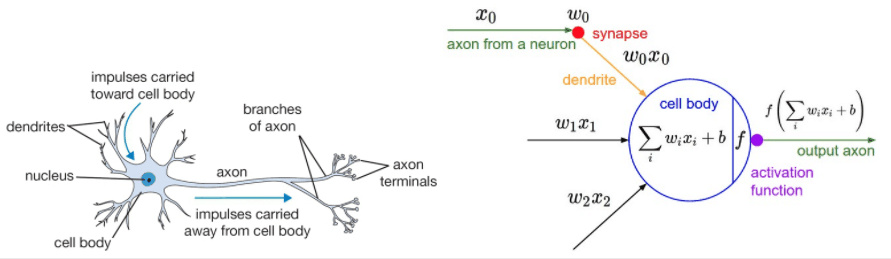左边是一张神经元的图片，神经元通过突触接受输入，然后通过神经激活的方式传输给后面的神经元。这对比于右边的神经网络，首先接受数据输入，然后通过计算得到结果，接着经过激活函数，再传给第二层的神经元。

所以前面讲的 logistic 回归模型和线性回归模型都可以看做是一个单层神经网络，而 logistic 回归中使用了激活函数 sigmoid。

神经网络使用的激活函数都是非线性的，每个激活函数都输入一个值，然后做一种特定的数学运算得到一个结果，下面举几个例子

### sigmoid 激活函数

σ ( x ) = 1 1 + e − x \sigma(x) = \frac{1}{1 + e^{-x}}

def Sigmoid(x):
return 1 / (1 + np.exp(-x))

x = np.arange(-10,10,0.1)
plt.plot(x, Sigmoid(x),clip_on=False)
plt.title('Sigmoid')### tanh 激活函数

t a n h ( x ) = 2 σ ( 2 x ) − 1 tanh(x) = 2 \sigma(2x) - 1

def Tanh(x):
return 2 * Sigmoid(2*x) - 1

plt.plot(x, Tanh(x))
plt.title('Tanh')### ReLU 激活函数

R e L U ( x ) = m a x ( 0 , x ) ReLU(x) = max(0, x)

def Relu(x):
return np.maximum(0, x)

plt.plot(x, Relu(x))
plt.title('Relu')## 神经网络的结构

神经网络就是很多个神经元堆在一起形成一层神经网络，那么多个层堆叠在一起就是深层神经网络，我们可以通过下面的图展示一个两层的神经网络和三层的神经网络可以看到，神经网络的结构其实非常简单，主要有输入层，隐藏层，输出层构成，输入层需要根据特征数目来决定，输出层根据解决的问题来决定，那么隐藏层的网路层数以及每层的神经元数就是可以调节的参数，而不同的层数和每层的参数对模型的影响非常大.

神经网络向前传播也非常简单，就是一层一层不断做运算就可以了，可以看看下面这个例子## 为什么要使用激活函数

激活函数在神经网络中非常重要，使用激活函数也是非常必要的，前面我们从人脑神经元的角度理解了激活函数，因为神经元需要通过激活才能往后传播，所以神经网络中需要激活函数，下面我们从数学的角度理解一下激活函数的必要性。

比如一个两层的神经网络，使用 A 表示激活函数，那么

y = w 2 A ( w 1 x ) y = w_2 A(w_1 x)

如果我们不使用激活函数，那么神经网络的结果就是

y = w 2 ( w 1 x ) = ( w 2 w 1 ) x = w ˉ x y = w_2 (w_1 x) = (w_2 w_1) x = \bar{w} x

可以看到，我们将两层神经网络的参数合在一起，用 w ˉ \bar{w} 来表示，两层的神经网络其实就变成了一层神经网络，只不过参数变成了新的 w ˉ \bar{w} ，所以如果不使用激活函数，那么不管多少层的神经网络， y = w n ⋯ w 2 w 1 x = w ˉ x y = w_n \cdots w_2 w_1 x = \bar{w} x ，就都变成了单层神经网络，所以在每一层我们都必须使用激活函数。

最后我们看看激活函数对神经网络的影响可以看到使用了激活函数之后，神经网络可以通过改变权重实现任意形状，越是复杂的神经网络能拟合的形状越复杂，这就是著名的神经网络万有逼近定理。

下面我们通过例子来感受一下神经网络的强大之处

## 二分类问题

import torch
import numpy as np
from torch import nn
import torch.nn.functional as F

import matplotlib.pyplot as plt
%matplotlib inline


## 决策边界

首先我们先定义一个决策边界的函数，这样可以方便我们后面画出我们的决策边界，这里我们是采用了plt.contourf来进行画，因为有时候我们的函数并不是一个线性函数，很难表示。

def plot_decision_boundary(model, x, y):
# Set min and max values and give it some padding
x_min, x_max = x[:, 0].min() - 1, x[:, 0].max() + 1
y_min, y_max = x[:, 1].min() - 1, x[:, 1].max() + 1
h = 0.01
# Generate a grid of points with distance h between them
xx, yy = np.meshgrid(np.arange(x_min, x_max, h), np.arange(y_min, y_max, h))
# Predict the function value for the whole grid
Z = model(np.c_[xx.ravel(), yy.ravel()]) # 得到所有的点的坐标
Z = Z.reshape(xx.shape)
# Plot the contour and training examples
plt.contourf(xx, yy, Z, cmap=plt.cm.Spectral) # 绘制等高线轮廓
plt.ylabel('x2')
plt.xlabel('x1')
plt.scatter(x[:, 0], x[:, 1], c=y.reshape(-1), s=40, cmap=plt.cm.Spectral)


这次我们仍然处理一个二分类问题，但是比前面的 logistic 回归更加复杂

## 随机生成数据

首先我们随机生成一些数据

np.random.seed(1)
m = 400 # 样本数量
N = int(m/2) # 每一类的点的个数
D = 2 # 维度
x = np.zeros((m, D))
y = np.zeros((m, 1), dtype='uint8') # label 向量，0 表示红色，1 表示蓝色
a = 4

for j in range(2):
ix = range(N*j,N*(j+1))
t = np.linspace(j*3.12,(j+1)*3.12,N) + np.random.randn(N)*0.2 # theta
r = a*np.sin(4*t) + np.random.randn(N)*0.2 # radius
x[ix] = np.c_[r*np.sin(t), r*np.cos(t)]
y[ix] = j

plt.scatter(x[:, 0], x[:, 1], c=y.reshape(-1), s=40, cmap=plt.cm.Spectral)## logistic 回归解决

x = torch.from_numpy(x).float()
y = torch.from_numpy(y).float()

w = nn.Parameter(torch.randn(2, 1))
b = nn.Parameter(torch.zeros(1))

optimizer = torch.optim.SGD([w, b], 1e-1)

def logistic_regression(x):

criterion = nn.BCEWithLogitsLoss()

for e in range(100):
out = logistic_regression(Variable(x))
loss = criterion(out, Variable(y))
loss.backward()
optimizer.step()
if (e + 1) % 20 == 0:
print('epoch: {}, loss: {}'.format(e+1, loss.data))

epoch: 20, loss: 0.7033562064170837
epoch: 40, loss: 0.6739853024482727
epoch: 60, loss: 0.6731640696525574
epoch: 80, loss: 0.6731465458869934
epoch: 100, loss: 0.6731461882591248


## 结果演示

def plot_logistic(x):
x = Variable(torch.from_numpy(x).float())
out = F.sigmoid(logistic_regression(x))
out = (out > 0.5) * 1
return out.data.numpy()

plot_decision_boundary(lambda x: plot_logistic(x), x.numpy(), y.numpy())
plt.title('logistic regression')可以看到，logistic 回归并不能很好的区分开这个复杂的数据集，如果你还记得前面的内容，你就知道 logistic 回归是一个线性分类器，这个时候就该我们的神经网络登场了！

## 神经网络解决二分类问题

# 定义两层神经网络的参数
w1 = nn.Parameter(torch.randn(2, 4) * 0.01) # 隐藏层神经元个数 2
b1 = nn.Parameter(torch.zeros(4))

w2 = nn.Parameter(torch.randn(4, 1) * 0.01)
b2 = nn.Parameter(torch.zeros(1))

# 定义模型
def two_network(x):
x1 = torch.mm(x, w1) + b1
x1 = F.tanh(x1) # 使用 PyTorch 自带的 tanh 激活函数
x2 = torch.mm(x1, w2) + b2
return x2

optimizer = torch.optim.SGD([w1, w2, b1, b2], 1.)

criterion = nn.BCEWithLogitsLoss()

# 我们训练 10000 次
for e in range(10000):
out = two_network(Variable(x))
loss = criterion(out, Variable(y))
loss.backward()
optimizer.step()
if (e + 1) % 1000 == 0:
print('epoch: {}, loss: {}'.format(e+1, loss.data))

def plot_network(x):
x = Variable(torch.from_numpy(x).float())
x1 = torch.mm(x, w1) + b1
x1 = F.tanh(x1)
x2 = torch.mm(x1, w2) + b2
out = F.sigmoid(x2)
out = (out > 0.5) * 1
return out.data.numpy()

plot_decision_boundary(lambda x: plot_network(x), x.numpy(), y.numpy())
plt.title('2 layer network')可以看到神经网络能够非常好地分类这个复杂的数据，和前面的 logistic 回归相比，神经网络因为有了激活函数的存在，成了一个非线性分类器，所以神经网络分类的边界更加复杂。

下一章传送门：Note13 反向传播算法

展开全文python 深度学习
• 在计算机上验证和测试多层神经网络的原理和算法实现，测试多层神经网络的训练效果，同时查阅相关资料。掌握多层神经网络的基本原理、掌握多层神经网络的算法过程、反向传播的算法过程； 三、实验内容 题1： 请阅读...

关于多层神经网络搭建部分的数学推导，我放在了实验总结部分，其中涉及到很多矩阵的运算，可以查看我的这篇文章简单了解一下： 矩阵求导之布局分析
关于为什么没有实验二的文章，大概是因为实验二不太像个正经实验…😅

# 一、实验要求

在计算机上验证和测试多层神经网络的原理和算法实现，测试多层神经网络的训练效果，同时查阅相关资料。

# 二、实验目的

1. 掌握多层神经网络的基本原理；
2. 掌握多层神经网络的算法过程；
3. 掌握反向传播的算法过程；

# 三、实验内容

## 1. 多层神经网络Python实现：

阅读和测试多层神经网络类代码，观察多层神经网络训练过程和结果，并对隐藏层Dense类和多层神经网络MLPClassifier类的代码进行详细注释。

### 1.1 导入所需的函数库

from sklearn import datasets
import numpy as np
import matplotlib.pyplot as plt
from sklearn.linear_model import Perceptron #感知机
from sklearn.neural_network import MLPClassifier #多层神经网络

from warnings import simplefilter
simplefilter(action='ignore', category=FutureWarning)

plt.rcParams['font.sans-serif'] = ['SimHei']
plt.rcParams['axes.unicode_minus']=False  # 用来正常显示负号


### 1.2 定义分界线绘制函数

'''分界线绘制函数'''
def plot_decision_boundary(model, X, y):
x0_min, x0_max = X[:,0].min()-1, X[:,0].max()+1
x1_min, x1_max = X[:,1].min()-1, X[:,1].max()+1
x0, x1 = np.meshgrid(np.linspace(x0_min, x0_max, 100), np.linspace(x1_min, x1_max, 100))
Z = model.predict(np.c_[x0.ravel(), x1.ravel()])
Z = Z.reshape(x0.shape)

plt.contourf(x0, x1, Z, cmap=plt.cm.Spectral)
plt.ylabel('x1')
plt.xlabel('x0')
plt.scatter(X[:, 0], X[:, 1], c=np.squeeze(y))


### 1.3 定义基类：Layer

'''定义一个基类：层Layer'''
class Layer:
def __init__(self):
pass
#前向计算
def forward(self, input):
return input
#反向传播
pass


### 1.4 定义激活函数层：Sigmoid

'''定义Sigmoid层'''
class Sigmoid(Layer):
def __init__(self):
pass

def _sigmoid(self,x):
return 1.0/(1+np.exp(-x))

def forward(self,input):
return self._sigmoid(input)

# 这里的input是：激活函数sigmoid的输入，即：z（z=X*W+b）
# 计算sigmoid函数（标量变元标量函数）对它的输入z（z=X*W+b）的偏导
# 返回：损失函数J对其输入a的偏导*sigmoid函数对其输入z的偏导 = 损失函数J对z的偏导


### 1.5 定义隐藏层：Dense

'''定义隐藏层'''
class Dense(Layer):
def __init__(self, input_units, output_units, learning_rate=0.1):
# 定义学习率
self.learning_rate = learning_rate
# 定义权重w（矩阵），行数是输入个数，列数是输出个数
# 每一个列向量就是一组输入对应当前输出的权重，几个输出就有几列
self.weights = np.random.randn(input_units, output_units)#初始化影响很大
# 定义偏差b（向量），长度等于输出个数
# 每一个分量就是一组输入对应当前输出的偏差，几个输出就有几个分量
self.biases = np.zeros(output_units)

'''前向积累，计算输出值'''
def forward(self,input):
# z=X*W+b，x为上一层的输出a_(i-1)，或最开始的输入
z = np.dot(input,self.weights)+self.biases
return z

'''反向传播，计算梯度'''

# 因为使用整体的损失对w求偏导，所以除以样本数量（input.shape）求均值

# 同理，使用整体的损失对b求偏导，所以求均值

# 利用梯度下降法优化当前层的w和b

# 返回J对当前层输入的梯度，用于前一层计算


### 1.6 定义多层神经网络：MLPClassifier

'''定义多层神经网络层'''
class MLPClassifier(Layer):
def __init__(self):
self.network = []
# 添加一个2输入，5输出的隐藏层
self.network.append(Dense(2,5))
# 为该隐藏层添加激活函数
self.network.append(Sigmoid())
# 添加一个5输入，1输出的隐藏层
self.network.append(Dense(5,1))
# 为该隐藏层添加激活函数
self.network.append(Sigmoid())

def forward(self,X):
# 列表存储中间计算结果
self.activations = []
input = X
# 正向循环遍历神经网络
for layer in self.network:
# 正向累积并存储中间值
self.activations.append(layer.forward(input))
# 将列表中存储的最后一个元素（前一次输出）作为下一次运算的输入
input = self.activations[-1]
# assert断言函数，不满足其后的条件则抛出错误，等价于 if not xxx：raise xxx
assert len(self.activations) == len(self.network)
return self.activations

'''本例中用于分界线绘制函数内调用predict方法'''
def predict(self,X):
# 正向累积，并获取最终输出
y_pred = self.forward(X)[-1]
# 根据输出结果实行二分类打标签
y_pred[y_pred>0.5]  = 1
y_pred[y_pred<=0.5] = 0
# 返回标签值
return y_pred

'''本例中没用上'''
def predict_proba(self,X):
logits = self.forward(X)[-1]
# 返回最终输出结果，而不是分类后的标签
return logits

'''具体训练方法，由train方法调用'''
def _train(self,X,y):
#先正向计算，再反向传播，梯度下降更新权重参数w，b
self.forward(X)
# 输入列表：layer_inputs，保存了每一层的输入，注意列表间的“+”是连接操作
layer_inputs = [X]+self.activations
# 最后一层的输出
logits = self.activations[-1]

# 这里的损失函数需要自己定义，这里用到最小二乘
loss = np.square(logits - y.reshape(-1,1)).sum()
# 损失函数对l最终输出（logits）的梯度值
# 反向循环遍历神经网络（反向传播）
for layer_i in range(len(self.network))[::-1]:
layer = self.network[layer_i]
# 计算损失函数对当前层输出（layer_inputs[layer_i]）的梯度
# 用该梯度可以得到损失函数对w和b的梯度，用于优化参数（在backward中进行了）
return np.mean(loss)

def train(self, X, y):
for e in range(1000):
# 神经网络迭代1000次，计算并查看每一次的损失是否变化
loss = self._train(X,y)
print(loss)
return self


### 1.7 生成训练集和测试集

# 生成散点簇数据集
x_train,y_train = datasets.make_blobs(n_samples=100, n_features=2, centers=2, cluster_std=1)
plt.scatter(x_train[y_train==0,0],x_train[y_train==0,1])
plt.scatter(x_train[y_train==1,0],x_train[y_train==1,1])
plt.show()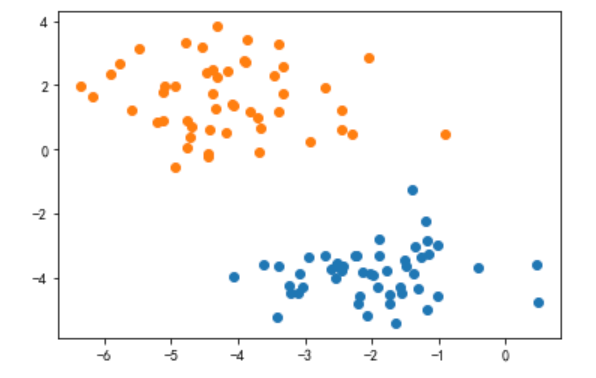### 1.8 训练并绘制分界线

MLP = MLPClassifier().train(x_train,y_train)

32.17454887891505
31.794027620971942
31.404196873755865
31.004962567950013

19.145328784895174
18.54282599092301
17.943890203632968

2.009292596684285
2.0019482522644507
1.9946533499084804
1.98740737422343

0.49032376234193314
0.48981025722326077
0.4892978159963806
0.488786435393049
plot_decision_boundary(MLP,x_train,y_train)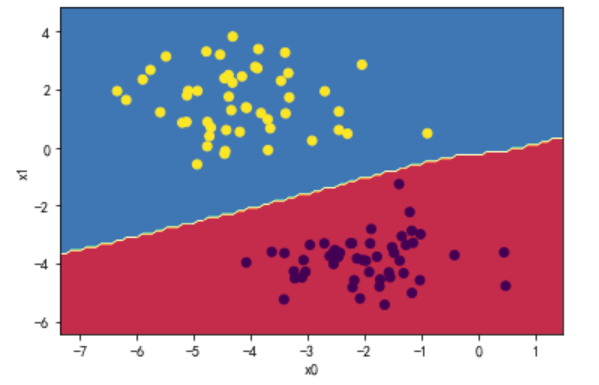## 2. 神经网络对层数和神经元数改进：

对多层神经网络改进，实现对moons数据的分类。

• 增加隐藏层神经元个数
• 增加隐藏层的层数

### 2.1 生成训练集和测试集

x_train,y_train = datasets.make_moons(n_samples=100,noise=0.2,random_state=666)
plt.scatter(x_train[y_train==0,0],x_train[y_train==0,1])
plt.scatter(x_train[y_train==1,0],x_train[y_train==1,1])
plt.show()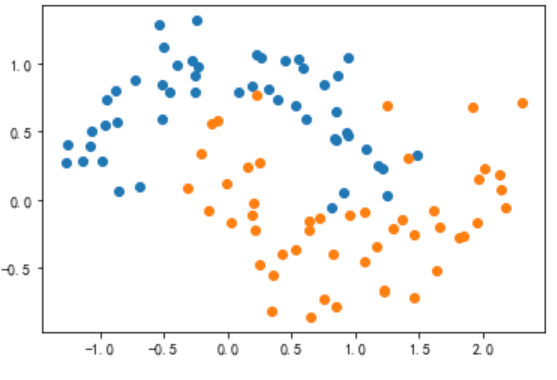### 2.2 增加神经网络层数和神经元

class MLPClassifier2(MLPClassifier):
def __init__(self):
self.network = []
# 添加一个2输入，6输出的隐藏层
self.network.append(Dense(2,6,0.5))
# 为该隐藏层添加激活函数
self.network.append(Sigmoid())
# 添加一个6输入，6输出的隐藏层
self.network.append(Dense(6,8,0.5))
# 为该隐藏层添加激活函数
self.network.append(Sigmoid())
# 添加一个6输入，4输出的隐藏层
self.network.append(Dense(8,4,0.5))
# 为该隐藏层添加激活函数
self.network.append(Sigmoid())
# 添加一个6输入，6输出的隐藏层
self.network.append(Dense(4,1,0.5))
# 为该隐藏层添加激活函数
self.network.append(Sigmoid())


### 2.3 训练并绘制分界线

MLP = MLPClassifier().train(x_train,y_train)

38.21754373561508

28.10775704700434

15.712500154068152

7.704956511113001

1.5864199654500957
plot_decision_boundary(MLP,x_train,y_train)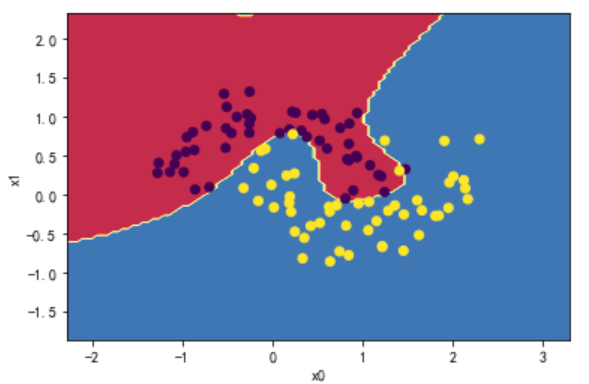## 3. 激活函数的替换和比较：

实现ReLU激活函数类，与Sigmoid激活函数类作比较，针对同样二分类问题训练的区别。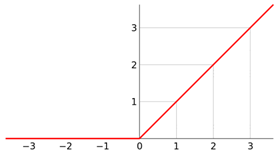### 3.1 定义激活函数层：ReLU

'''定义Relu层'''
class ReLU(Layer):
def __init__(self):
pass

def forward(self,input):
return np.maximum(0,input)

# 计算relu函数对其输入z（z=X*W+b）的偏导
# 如果输入大于0，导数为1；否则为0。
# 返回：损失函数J对其输入a的偏导*relu函数对其输入z的偏导 = 损失函数J对z的偏导


### 3.2 比较损失下降速度

class MLPClassifier(Layer):
def __init__(self):
self.network = []
# 添加一个2输入，5输出的隐藏层
self.network.append(Dense(2,5))
# 为该隐藏层添加激活函数
self.network.append(ReLU())
# 添加一个5输入，1输出的隐藏层
self.network.append(Dense(5,1))
# 为该隐藏层添加激活函数
self.network.append(Sigmoid())

• 左为隐藏层1使用sigmoid激活函数；右为隐藏层1使用relu激活函数；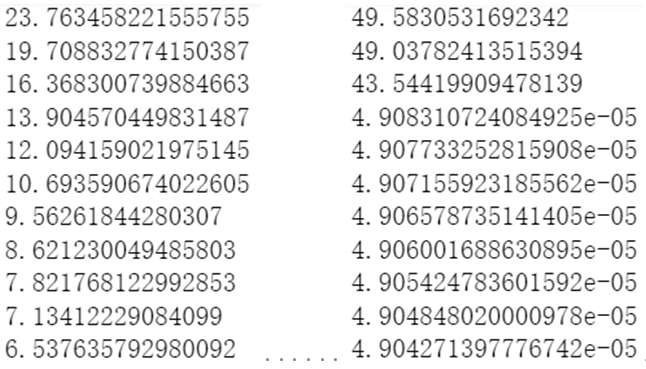可以看到使用relu激活函数显著加快了学习速率，损失在第四次迭代时呈断崖式下降。

# 四、实验总结

• 关于题一的数学推导如下：• 关于上面的推导中采用了分子布局的方式进行整体的计算，结果该式出现与数学上矩阵求导计算顺序不符的问题： δ J ( A 2 , Y ) δ X T \frac{\delta{J(A_{2},Y)}}{\delta{X^{T}}}

• 不仅如此，若是采用分母布局对整体进行推导，虽然矩阵 X X 求得的偏导没问题，但又导致了 J ( A 2 , Y ) J(A_{2},Y) W W B B 的偏导不满足数学上矩阵求导的计算顺序 δ J ( A 2 , Y ) T δ W ， δ J ( A 2 , Y ) T δ B \frac{\delta{J(A_{2},Y)^{T}}}{\delta{W}}，\frac{\delta{J(A_{2},Y)^{T}}}{\delta{B}}

• 初步分析应该是输入矩阵 X X 的设计没有满足 Z = X T W + B Z=X^{T}W+B ，再加之矩阵运算的复杂性，求导过程中需要进行一定变更，否则就会出现上述矛盾。这一问题的答案将在日后推导，这个实验目前耗时太多了…

展开全文深度学习 算法 矩阵 python
• 作用：引入神经网络 2、matplotlib.pyplot包 作用：绘图 3、numpy包 作用：矩阵的相关运算 代码如下： #首先导入tensorflow包、numpy包、matplotlib包 import tensorflow as tf import numpy as np import人工智能 tensorflow
• 构建一个至少含有1-2 层隐藏层的神经网络模型，解决手写的0-9 十个手写 数字的识别问题。神经网络模型构建过程中需要注意的几点： （1）数据集采用MNIST 阿拉伯数字手写体数据集。 （2）模型输入层的节点个数的设计...python
• 基于多层前馈神经网络的航天器在线故障检测系统设计.pdf
• 一、多层神经网络 1、多层神经网络数学模型 2、数学模型中的非线性函数fai 1）非线性函数fai存在的意义 2）非线性函数fai具体是什么？ 3、多层神经网络与单层神经网络的区别与改进 1）单层神经网络数学模型 2...
• 基于多核处理器的多层感知神经网络设计和实现.pdf
• 推导前向多层神经网络的学习算法，以及BP学习算法中几个值得注意的问题，设计网络学习过程的步骤
• 此篇的任务是：构建深度神经网络所需要的函数，为下一任务的“构建用于图像分类的深度神经网络”做准备。 任务所需要的资料可以在末尾参考链接第一条找到。 我用的环境是天池的notebook 构建单层神经网络的前向后向...
• 本研究旨在通过人工神经网络建立降雨径流模型，以填补卡夫里河流域伊特水文站流量数据系列的空白。 利用流量蒸散数据建立了具有两个条目（每月平均降雨量和蒸散量）和出口（流量）的前馈的多层感知器。 神经网络...
• 本处用一个多层前向神经网络，采用反向传播算法依据控制要求实时输出Kp、Ki、Kd，依次作为PID控制器的实时参数，代替传统PID参数靠经验的人工整定和工程整定，以达到对大迟延主气温系统的良好控制。对这样一个系统在...
• 用Tensorflow实现多层神经网络 觉得有用的话,欢迎一起讨论相互学习~Follow Me 参考文献 Tensorflow机器学习实战指南 源代码请点击下方链接欢迎加星 ReLU激活函数/L1范数版本 Sigmoid激活函数/交叉熵...Tensorflow
• 隐含层、学习率、阈值函数均可灵活修改，如果需要，可以修改宏头实现任意层的bp网络的搭建和学习，程序自动生成w.txt记录学习的权值并在屏幕上显示，便于找寻权值学习的规律，主要参考了别人神经网络类的设计，...
• 针对心电信号检测中主要存在的几类噪声，利用BP神经网络的非线性映射能力设计了基于BP神经网络的非线性滤波器，实现了对心电信号中工频干扰、基线漂移、电极移动等噪声的滤除。经仿真与实验验证表明，该方案对于工频...
• 将神经网络总体平均误差作为目标函数,以待求的神经网络权值和阈值作为设计变 量,通过设计变量合理排序与分配,提出多隐层多层神经网络权值和阈值计算的高精度真实共轭 梯度最优化算法。与 BP算法和梯度优化算法相比,...
• 多层神经网络（MNN）和傅立叶级数展开（FSE）被组合到新颖的逼近器中进行建模系统中的每个不确定性。 动态表面控制（DSC）方法和积分型李雅普诺夫函数（ILF）技术组合设计控制算法。 所有闭环的极限一致有界信号...研究论文
• ## 多层神经网络ANN

千次阅读 2016-08-14 22:32:23
本文基于 cnblog学习总结，基于的博客地址如下： http://www.cnblogs.com/ronny/p/ann_02.html在实际应用过程中...多层神经网络是由多个层结构组成的网络系统，他的每一层都是若干个神经元结点构成，该层的任意一个结点
• 从广义上说深度学习的网络结构也是多层神经网络的一种。传统意义上的多层神经网络是只有输入层、隐藏层、输出层。其中隐藏层的层数根据需要而定，没有明确的理论推导来说明到底多少层合适。而深度学习中最著名的卷积...深度学习
•深度学习
• Python 多层BP神经网络的实现及应用 神经网络 前向传播（Forward propagation） 反向传播（Error Back propagation） Python代码实现BP神经网络 在深度学习中，其中一种网络架构是前向传播——反向传播，本文就讲解...反向传播 python
• 在各种神经网络中，多层前馈神经网络具有很强的信息处理能力，由于其采用BP算法，因此也称为BP神经网络。采用BP神经网络模型能完成图像数据的压缩处理。在图像压缩中，神经网络的处理优势在于：巨量并行性；信息处理...
• 本项目手打了一个简单的BP神经网络。网络有三层——输入层、隐含层、输出层，使用sigmoid函数作为激活函数，通过反向梯度更新网络权值。同时手打一个PCA降维函数，完成数据的预处理。项目中包含两个数据集——...
• 主要为大家详细介绍了基于循环神经网络(RNN)实现影评情感分类，具有一定的参考价值，感兴趣的小伙伴们可以参考一下
• 多层神经网络对于多层神经网络的训练，delta规则是无效的，因为应用delta规则训练必须要误差，但在隐含层中没有定义。输出节点的误差是指标准输出和神经网络输出之间的差别，但训练数据不提供隐藏层的标准输出。真正......